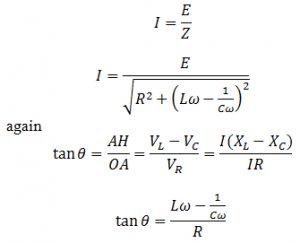# AC Through Inductor, Capacitor And Resistor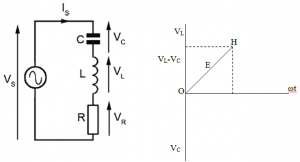A pure inductor, an ideal capacitor and a pure resistor are connected in series to an alternating current source. Since the components are connected in series,same current flows across each of them while each of them suffer different potential drop.
Let E be the rms value of applied emf and I be the rms value of the current flowing in the circuit. Let VL , VC and VR be the potential drop across L, C and R respectively.
The potential drop across inductor is: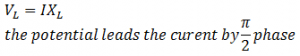The potential drop across capacitor is: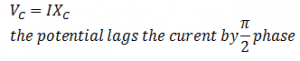The potential drop across resistance is: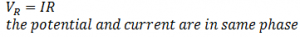From the fig the resultant OH is given by: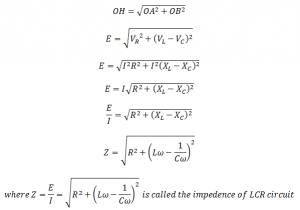This is the3 impedance of LCR circuit. It is the effective opposition offered by LCR circuit for the flow of current.